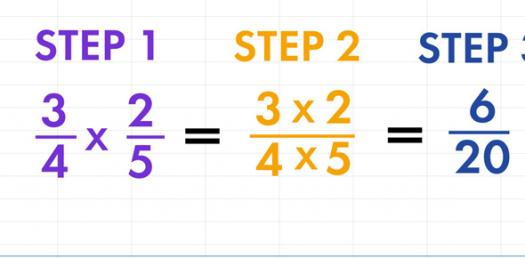# B - Multiplying Fractions

Approved & Edited by ProProfs Editorial Team
At ProProfs Quizzes, our dedicated in-house team of experts takes pride in their work. With a sharp eye for detail, they meticulously review each quiz. This ensures that every quiz, taken by over 100 million users, meets our standards of accuracy, clarity, and engagement.
| Written by Deborah Eberhardt
D
Deborah Eberhardt
Community Contributor
Quizzes Created: 6 | Total Attempts: 2,533
Questions: 5 | Attempts: 249Settings.

• 1.

### 2/6*3/5

• A.

1/5

• B.

2/10

• C.

2/3

A. 1/5
Explanation
To find the product of two fractions, we multiply the numerators together and the denominators together. In this case, the numerator is 2 multiplied by 3, which equals 6. The denominator is 6 multiplied by 5, which equals 30. Therefore, the simplified fraction is 6/30, which reduces to 1/5.

Rate this question:

• 2.

### 9/3*5/8

• A.

1 4/45

• B.

3 2/34

• C.

33

A. 1 4/45
Explanation
The given expression is a multiplication of two fractions. To simplify, we multiply the numerators together (9 * 5) and the denominators together (3 * 8). This gives us the fraction 45/24. Simplifying this fraction, we divide both the numerator and denominator by their greatest common divisor, which is 3. This results in the fraction 15/8. However, since the numerator is greater than the denominator, we can divide 15 by 8 to get a whole number and a remainder. Dividing 15 by 8 gives us 1 with a remainder of 7. Therefore, the final answer is 1 7/8, or in mixed number form, 1 4/45.

Rate this question:

• 3.

### 3/4*5/2

• A.

3/6

• B.

1 7/8

• C.

2/5

B. 1 7/8
Explanation
The given expression is a multiplication of two fractions. To multiply fractions, we multiply the numerators together and the denominators together. In this case, the numerator is 3 * 5 = 15, and the denominator is 4 * 2 = 8. So, the result is 15/8. However, we can simplify this fraction by dividing both the numerator and denominator by their greatest common divisor, which is 1. Dividing 15 by 8 gives us a whole number quotient of 1 and a remainder of 7. Therefore, the simplified fraction is 1 7/8.

Rate this question:

• 4.

### 1/8*7/9

• A.

12/87

• B.

7/72

• C.

3/72

B. 7/72
Explanation
The given expression is a multiplication of two fractions. To multiply fractions, we multiply the numerators together and multiply the denominators together. In this case, the numerator is 1 times 7 which equals 7, and the denominator is 8 times 9 which equals 72. Therefore, the simplified fraction is 7/72.

Rate this question:

• 5.

### 5/7 * 5/7

• A.

25/7

• B.

35/49

• C.

25/49

• D.

1Back to top